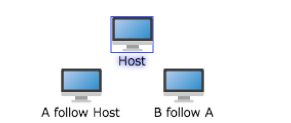Light High Efficiency Graph Component

# Node Follow

Node(A) can follow another node (B) to move. In this case, node B is called host node. Node A is called follower

• host - host node
• clearFollowers - clear following node

#### Example

Set node A to follow Host, and node B to follow A

``````var host = graph.createNode("Host");
var a = graph.createNode("A follow Host", -50, 50);
a.host = host;
var b = graph.createNode("B follow A", 50, 50);
b.host = a;
``````

Operation effect: